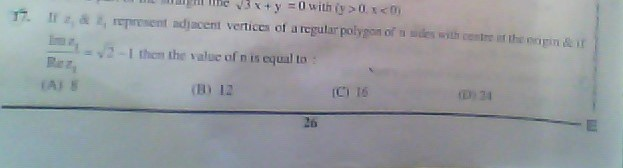# the area of triangle whose vertices are the roots z3+iz2+2i5 years ago
Dear Ashish

iven cubic equation is

z3 + iz2 + 2i = 0

Now put z = i in LHS

i3 + i*i2 + 2i

= i*i2 + i*i2 + 2i

= -i -i +2i                    (since i2 = -1)

= -2i + 2i

= 0

= RHS

So z =i is a factor of given eqaution

Now when we divide  z3 + iz2 + 2i by z-i, we get other two factors 1-i and -1-i.

So three roots are i , 1-i, -1-i.

Now vertices of triangle are (0, 1), (1, -1), (-1, -1).

Now are of triangle is defined as

|0  1  1 |

(1/2)* |1 -1  1|

|-1 -1 1|

= (1/2)({0 -1(1+1) + 1(-1-1)}

= (1/2)*(-2-2)

= -4/2

= -2

By taking positive sign

Area of the traingle is 2 unit2

Regards